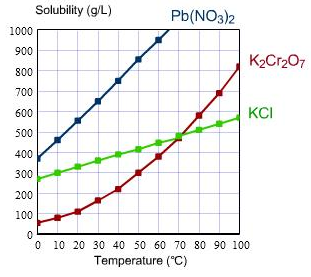# Problem: Here are the solubilities of some salts in water. For each salt, determine how much will remain undissolved if 500 g is mixed into a liter of pure water at 40°C. One significant figure is sufficient in each answer.

###### FREE Expert Solution###### Problem Details

Here are the solubilities of some salts in water.For each salt, determine how much will remain undissolved if 500 g is mixed into a liter of pure water at 40°C. One significant figure is sufficient in each answer.